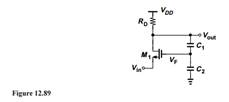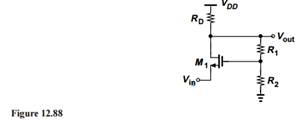### Create an Account

Home / Questions / Repeat Problem 25 for the topology of Fig 12 89 Assume C1 and C2 are very small and negle...

# Repeat Problem 25 for the topology of Fig 12 89 Assume C1 and C2 are very small and neglect other capacitances Problem 25 Consider the feedback circuit shown in Fi 12 88 where

Repeat Problem 25 for the topology of Fig. 12.89. Assume C1 and C2 are very small and neglect other capacitances.Problem 25

Consider the feedback circuit shown in Fig. 12.88, where R1 + R2 ≫ RD. Compute the closed-loop gain and I/O impedances of the circuit. Assume  λ ≠ 0.Apr 24 2020 View more View LessSubscribe To Get Solution